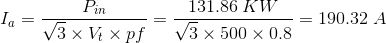# MCQs on Electrical Machines

##### Page 75 of 114. Go to page 1 2 3 4 5 6 7 8 9 10 11 12 13 14 15 16 17 18 19 20 21 22 23 24 25 26 27 28 29 30 31 32 33 34 35 36 37 38 39 40 41 42 43 44 45 46 47 48 49 50 51 52 53 54 55 56 57 58 59 60 61 62 63 64 65 66 67 68 69 70 71 72 73 74 75 76 77 78 79 80 81 82 83 84 85 86 87 88 89 90 91 92 93 94 95 96 97 98 99 100 101 102 103 104 105 106 107 108 109 110 111 112 113 114
01․ The synchronous motor can be started by
coupling it with a DC compound motor.
coupling it with a small AC induction motor called the pony motor.
providing damper winding in its rotor circuit.
any of the above methods.

Under construction.

02․ Under which of the following conditions hunting of synchronous motor is likely to occur ?
Over-excitation.

Under construction.

03․ A 500 V, 120 KW, 50 Hz, star connected synchronous motor operating at 0.8 power factor leading has full load efficiency of 91%. The armature resistance is equal to 0.08Ω. The magnitude of armature current is nearly equal to
127 A.
173 A.
190 A.
240 A.

Thw input power is Pin = Pout / φ· = 120 KW/0.91 = 131.86 KW. Armature current is given by,04․ For a practical synchronous motor the pull out torque will occur when the torque angle is nearly equal to
0°.
30°.
45°.
75°.

The maximum torque i.e. pull out torque will occur at θ = 90°. But this is possible for the motor having smooth rotors. As most practical motors have salient poles, so the pull out torque occurs at a torque angle about to 75°.

05․ If the mechanical angle ÃŽÂ± between the stator and rotor poles increases, then the stator current
Decreases.
Increases.
Remains unchanged.
None of above.

At no load the axis of stator and rotor poles concide. As the motor is loaded the rotor poles falls slightly behind the stator poles, while running at synchronous speed. The increased power is drawn from AC source (Stator Winding). Thus the stator current increases.

06․ In the V curve shown in figure below for a synchronous motor, the parameter of Y and X coordinates are, respectively
Torque and field current.
Armature current and field current.
Power factor and field current.
Armature current and torque.

When the magnitude of the armature current is plotted as a function of the excitation current for various load conditions, we obtain a set of nested curves, so called V curves.

07․ In synchronous generator a synchronous dead load (lamp, furnaces etc) has no ____________ hence it has no natural frequency of oscillation.
restoring torque.
mechanical rotatory system.
synchronizing power co efficient.
damping torque.

Under construction.

08․ In diesel engine, gas engine type of machine contains ___________________ pole type of alternator.
cylindrical.
salient.
non uniform cylindrical.
none of these.

Under construction.

09․ What is the frequency of a alternator, if P = number of poles and N = revolution made per second?
PN / 2 Hz.
120 / PN Hz.
120N / P Hz.
120P / N Hz.

If a two poles alternator rotates one complete rotation, it produces one cycle of generated voltage. If a P poles alternator rotates one complete rotation, it produces P/2 cycles of generated voltage. If a P poles alternator rotates N complete rotations, it produces NP/2 cycles of generated voltage. As the N is the rotation made per second, the frequency that is number of cycles produced during one second, of the generated voltage would be NP/2 Hz.

10․ In alternator, the rotary part is
armature.
core.
magnetic field poles.
none of these.

Basically, the alternators have the field winding in its rotary part and the armature windings in its stationary part. We know that the field winding produces the magnetic fields and the other name of the field winding is magnetic field poles. Hence, the rotary part of the alternator is magnetic field poles.

<<<7374757677>>>